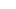Wooden Shoes
Knots & Climbing

# Figure eight bend

## What is the Figure Eight Bend?

The Figure Eight bend is a bend knot that can be used to tie two ropes together. The Figure Eight bend is safe to climb on but it is a bit bulky. The Figure Eight bend or Flemish bend is easy to tie and easy to remember. It is technically a Figure eight follow through so if you learn that one you just kill two birds with one stone. And the Figure eight follow through is a figure eight stopper knot that is retraced.

## Remarks

The Figure Eight Bend is also known under the name of Flemish Bend. Bends are the name for a type of knot that is used two join two ropes together. The Figure Eight bend works best with two ropes of equal type and diameter. The Figure Eight bend is relatively easy to untie even after it has been loaded.## FAQ Figure eight bend

### How do you tie two ropes together?

You can tie two ropes together with a knot type that is know as a bend. For example the Figure Eight bend. But there are many other types of bends that can be used for this purpose.

### How safe is the Figure Eight Bend

The Figure Eight bend is very save due through its non slip nature. All types of figure eight knots have been used for decades in climbing so they have proven them self over and over again in different situations.

### What is the Figure Eight Bend used for?

The Figure Eight bend is a so called Bend. Bends are a type of knot that are used to tie two ends of rope together.

### How tie the Figure Eight Bend?

To tie the Figure Eight Bend you first tie a regular figure eight knot and then retrace it with another rope. You can also watch our tutorial and learn by seeing the Figure Eight bend being tied https://youtu.be/Hrmdtr9RzqM